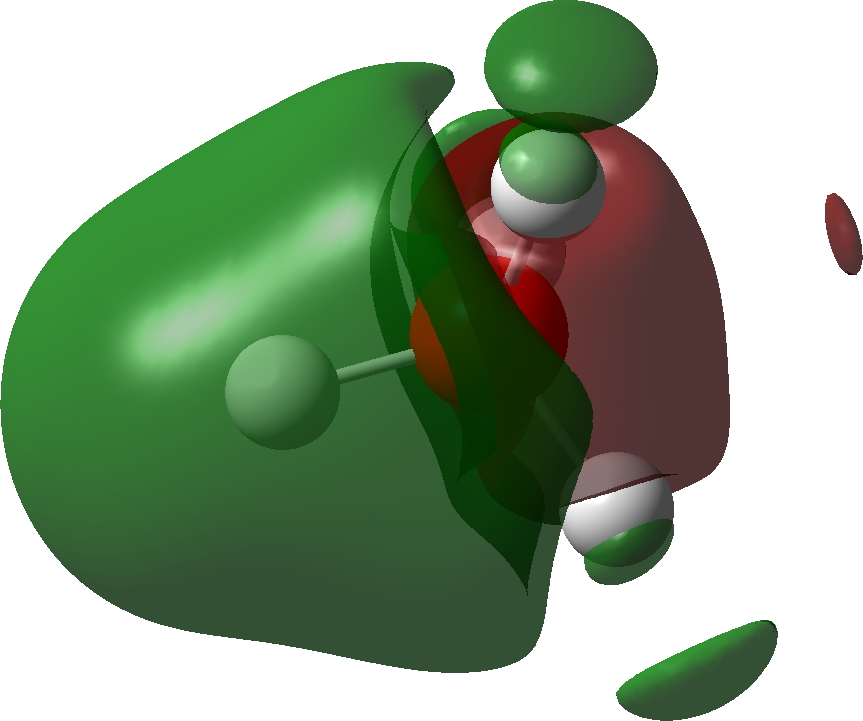## Ammonide: an alkalide formed from ammonia and resembling an electride.Alkalides are anionic alkali compounds containing e.g. sodide (Na), kalide (K), rubidide (Rb) or caeside (Cs). Around 90 examples can be found in the Cambridge structure database (see DOI: 10.14469/hpc/3453  for the search query and results). So what about the ammonium analogue, ammonide (NH4)? A quick search of Scifinder drew a blank! So here I take a look at this intriguingly simple little molecule.

It can be formed by adding two electrons to the ammonium cation; NH4+ + 2e ↠ NH4. One might be encouraged to do this since the LUMO (lowest unoccupied molecular orbital, below) of the ammonium cation has A1 symmetry and so can accept two electrons without the penalty of Jahn-Teller distortions. These electrons will apparently expand the valence electron “octet” around the nitrogen from 8 to 10; a hypervalent species then?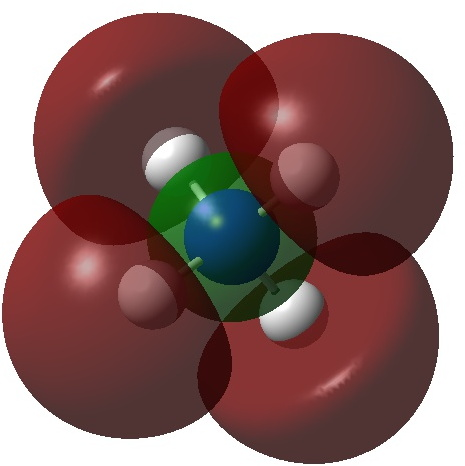So what are the (calculated) properties of NH4? The energy of the now HOMO (highest occupied molecular orbital) at the ωB97XD/Def2-TZVPPD/solvent=water level is -3.6eV, a respectable electron affinity (the additional electrons are said to be bound). More insight can be obtained from the NBO analysis, which produces localized versions of the molecular orbitals. There are four equivalent NBOs, one of which is shown below.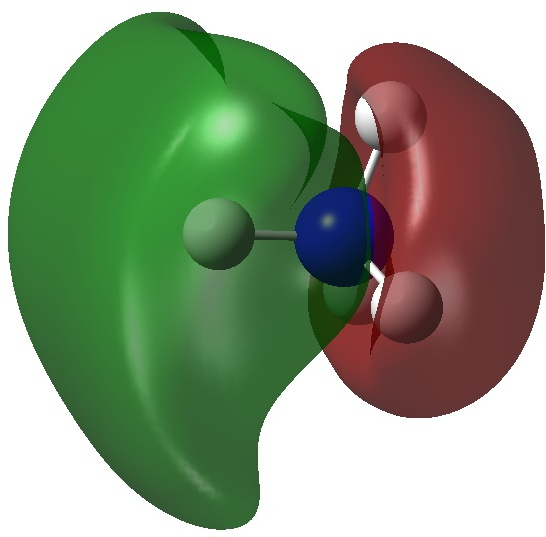Each is bonding along one H-N bond, mildly anti-bonding along the other three N-H bonds, but again bonding in the H-H regions! This matches the observations made earlier that when more electrons are pumped into normally valent main group molecules, they will occupy the antibonding levels. This is accompanied by a reduction in the bond orders associated with the central atom. In this case, the N-H bond orders are reduced from 0.79 to 0.602 and the total bond index at the nitrogen is reduced from 3.16 to 2.408. The bond index at hydrogen is at first sight increased from 0.79 to a surprising 1.0003, but this is explained because the H-H bond orders are 0.1328 (three for each H), which brings the H index up to 1.0. The N-H vibration (A1 symmetric) is 3466 cm-1 for NH4+  which is reduced to 2659 for NH4.

So it appears that adding two electrons to the ammonium cation induces H-H bonding! More insight can be obtained from an ELF analysis of the electron density basins.The above shows four attractors (as they are called) centered at the hydrogen nuclei, with 2.053e each (4*2.053 = 8.212e). The remaining ~2e are located in basins (green) centered at two different types of attractors. One is along the axis of each N-H bond and exo to it (0.316e) and the other sits on top of any set of three hydrogens (0.103e), 1.68e in total. The value of the ELF function at the attractor is shown above. You should realize that ELF=1.0 corresponds to perfectly localized electrons (for which the kinetic energy density is zero) and ELF=0.5 would correspond to a free-electron gas. The ELF value of e.g. 0.77 is getting close to an electron gas, and in fact corresponds to what we call an electride.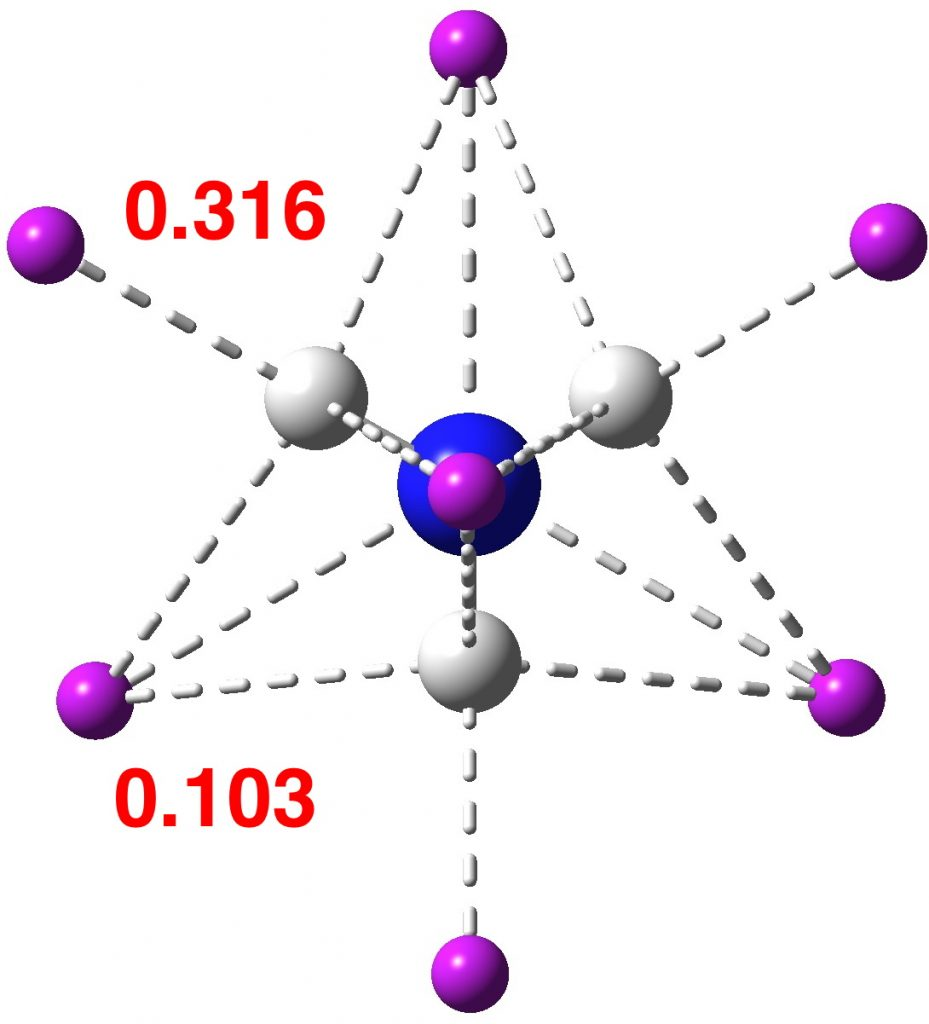So, the nitrogen valence shell electron octet is not actually exceeded! The additional two electrons in ammonide sit beyond the nitrogen, in H-H regions. Whether or not it is a viable species for detection remains to be established, but even its computed bonding properties have proved interesting and it deserves to join the alkalide family.

Postscript

Ammonide exists in a shallow well in the potential energy surface, shown below, with the dissociation to ammonia and hydride anion being exothermic.The intrinsic reaction coordinate shows one interesting feature at  IRC ~-1.1 which corresponds to repulsion between the hydride and the lone pair of the nitrogen atom resulting in inversion of configuration during the latter stages of the IRC.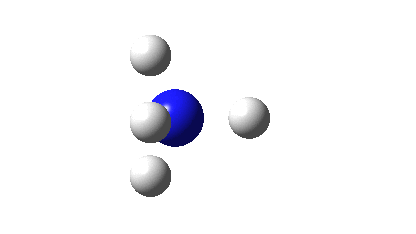FAIR data collection; 10.14469/hpc/3455. Perhaps unsurprisingly, these values are somewhat basis set dependent. Thus a ωB97XD/Def2-QZVPPD/Water calculation gives the following values: bond index at N, 1.998, N-H bond index, 0.4995, H-H bond index 0.1689, H bond index 1.0062, total Rydberg population, 0.2738, ν(A1) 2686 cm-1. The ELF basins are H, 2.039, exo-basins 0.282 and 0.141 (total 1.692). The improved basis set better describes the diffuse regions beyond the N-H bonds.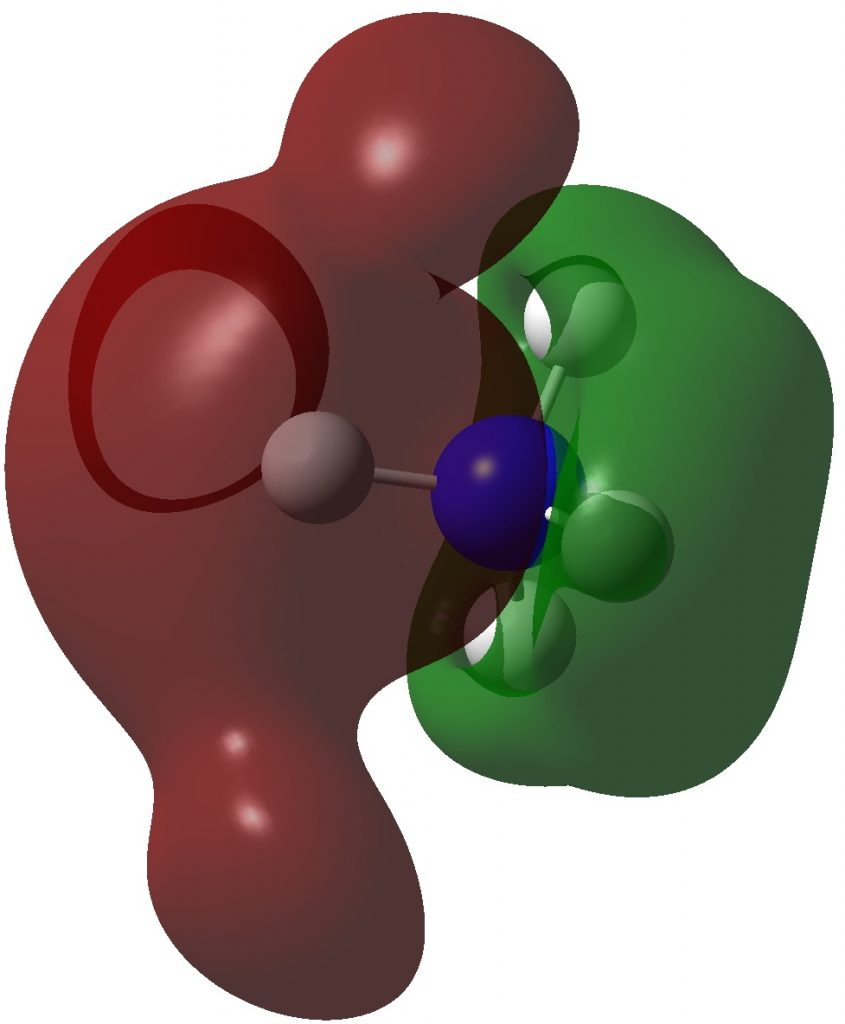### 2 Responses to “Ammonide: an alkalide formed from ammonia and resembling an electride.”

1.Mike Turner says:

A rough calculation suggests H2 + (NH2) are the thermodynamically preferred dissociation products. Is there no transition state leading to these products?

2.Henry Rzepa says:

Partially in response to the question above, here are some results for OH4, which is isoelectronic to NH4.

This species is not a stable minimum, but has a three-fold degenerate imaginary vibration (ν 1527i cm-1) corresponding to shortening two H-H distances and lengthening the other two.

The Wiberg bond index at O is 1.237, whilst the OH bond orders are 0.273 and the H-H bond orders 0.240. The Rydberg occupancy is only 0.105e.

The highest energy occupied NBO is shown below and as with ammonite, it is bonding in one OH region and in the H-H regions.